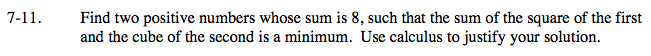### Home > CALC > Chapter 7 > Lesson 7.1.1 > Problem7-11

7-11.

Find two positive numbers whose sum is 8, such that the sum of the square of the first and the cube of the second is a minimum. Use calculus to justify your solution. Homework Help ✎Let y = a² + b³
Use the fact that a + b = 8 to rewrite y with only one variable.

Optimize! Find y' and set it equal to 0.

Be sure to answer the question completely. In other words: What is a? What is b?